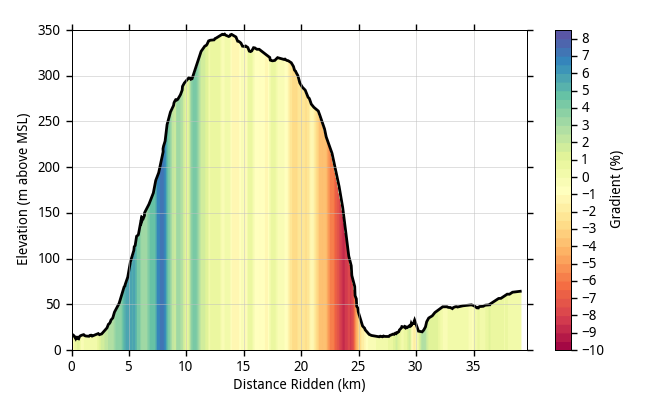# Help needed on specific plot

Hi,
I have the exterior shape of a nozzle given by S(x). Now what I want to
do is first reflect it with respect to the y=0 axis (easily achieved by
-S(x) right?) and then map each x node's value of an array (say density)
to a color that would fill the vertical space between S(x) and -S(x). In
other words I need something like the matplotlib.pyplot.fill_between
method using x, S(x) and -S(x) but where the fill color would be
relative to a third array (say density again) at each point x.
Thanks!

···

--
Hugo Gagnon
--
Hugo Gagnon

Hugo,

I don't have time to really explain how to do this, so instead I'm just going to send some code I made that I think might be very similar to what you're looking for.
http://dpaste.com/161843/

I've also attached some data so you can run the code.

Good luck,
-paulelevation.csv (63 KB)

···

-----Original Message-----
From: matplotlib@…2990… [mailto:matplotlib@…2990…]
Sent: Friday, February 19, 2010 2:16 PM
To: matplotlib-users@lists.sourceforge.net
Subject: [Matplotlib-users] Help needed on specific plot

Hi,
I have the exterior shape of a nozzle given by S(x). Now what I want to
do is first reflect it with respect to the y=0 axis (easily achieved by
-S(x) right?) and then map each x node's value of an array (say density)
to a color that would fill the vertical space between S(x) and -S(x). In
other words I need something like the matplotlib.pyplot.fill_between
method using x, S(x) and -S(x) but where the fill color would be
relative to a third array (say density again) at each point x.
Thanks!
--
Hugo Gagnon
--
Hugo Gagnon

-------------------------------------------------------------------------
-----